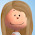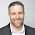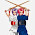## Friday, March 13, 2015

### I Hate the Pyramid

As a teacher with a strong background in math and physics, I have always hated - nay, LOATHED the pyramid. You may know of it as the magic triangle, or sometimes as a "formula circle." I hate them all.

If you have never come across such a device, it is simply a way of determining how to solve for any of three variables in an equation where two of the variables are multiplied to give the third value. In the first pyramid above, if you wanted to predict volume (V) and were given mass (m) and density (d), you would use your thumb to cover up the V in the pyramid (what you want to predict). Doing so leaves m over d - indicating you calculate mass divided by density.

Want to determine mass? Cover the m, and you see that you would multiply density and volume, since they are side-by-side.

Is it easy? Yes. And that's why many students (and teachers) gravitate toward using them. My issue, though, is that their usage relies on memorization (you have to get the pyramid right before you can use it), and it removes algebra from the process. Sure, a pyramid works very well for "rearranging" an equation like V = IxR, but what about SA = πrs + πr^2? And don't even get me started on the complete lack of unit analysis...

When I was actively teaching concepts like Ohm's Law (in the circle, above), I never mentioned the pyramid, and made all my students rearrange the equations using opposite operations. I've always insisted that they practice the "hard" way, to better prepare them for the day they need to solve more complex equations. I've even been known to "boo" loudly (with a smile!) if a pyramid ever showed up on a student's work.

But now I'm letting the students choose how they learn concepts like Ohm's Law, and even though the majority of the resources I provide (including the textbook) show no sign of the pyramid, lo and behold, the dang things are showing up EVERYWHERE.

Because I'm emphasizing that each student learns his or her own way, I find I can't - and I don't want to - fault the students for using them. I don't encourage the use of pyramids - I won't even mention pyramids in a mini-lesson, and if a student asked me how to solve for a variable, I'll walk them through using opposite operations. But if the students have independently found a method that allows them to master the learning goal, then more power to them! Right?

Incidents like these are forcing me to step back and really focus on the actual learning goals presented to my students. I am asking them to accurately predict electrical resistance when given voltage and current, NOT asking them to correctly rearrange an equation. In that sense, it doesn't matter how they do it - they could even use trial and error - as long as they eventually do.

I'm not 100% sure this is the correct way to proceed, and I'm finding the algebra a tough thing to let go of. I feel my students might be gaining in the short term (by accomplishing the learning goal), but losing in the long term (less rigorous practice). What do you think?

1.2.1.